# Summarize Attributes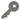Available in big data analytics.

The Summarize Attributes tool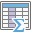summarizes like field values to generate a summary table. The resulting layer displays the count of features summarized as well as any additional statistics that have been specified.

## Workflow diagram## Example

Tornadoes and hurricanes are some of the most destructive types of storms in the United States. You want to analyze property damage and financial loss for tornadoes and hurricanes to compare how their impact differs. You have access to tornado and hurricane data across the United States in a single dataset and you want to summarize all the information to see a summary of values for all hurricanes and a summary of values for all tornadoes. You can summarize your data using the type of storm to determine the statistics for each storm type.

## Usage notes

• The Summarize Attributes tool is a tabular analysis tool, not spatial. The output table will consist of fields containing the result of the statistical operation.
• Using the Fields parameter, you can optionally specify one or more fields to summarize by or summarize all features. When you summarize by a single field, statistics are calculated for each unique attribute value. When you summarize by multiple fields, statistics are calculated for each unique combination of attribute values.
• The output of this tool will always include a count of the number of features that have been summarized.
• Additional statistics can be calculated using the Summary Fields parameter. The summary fields statistics available depend on the field type you are summarizing. A string attribute field can use the statistics any, count, and count distinct. A numeric attribute field can use the statistics any, count, count distinct, sum, sum of squares, min, max, range, variance, and standard deviation. A date attribute field can use the statistics any, count, min, max, and range.

## How Summarize Attributes works

The following describe how the Summarize Attributes tool works.

### Equations

Variance is calculated using the following equation:

Standard deviation is calculated as the square root of the variance.

### Calculations

Input layers are summarized into groups with matching field values. The results are tabular, so they cannot be visualized on your map.

The tables below illustrate the statistical calculations of a layer that is summarized using like field values. The VO2 field was used to calculate the numeric statistics (Count,Sum, Minimum, Maximum, Range, Mean, Standard Deviation, and Variance) for the layer. The Rating field was used to calculate the string statistics (Count and Any) for the layer.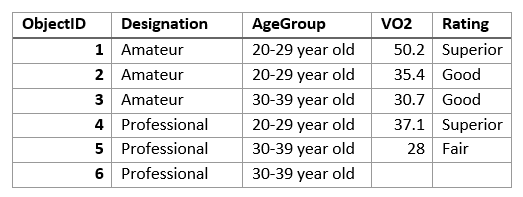The table above was summarized on the Designation field, and the VO2 field was used to calculate the numeric statistics (Count,Sum, Minimum, Maximum, Range, Mean, Standard Deviation, and Variance) for the layer. The Rating field was used to calculate the string statistics (Count and Any) for the layer. This result is a table with two features, representing the distinct values of Designation.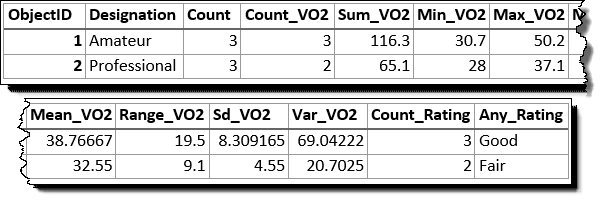The following table represents how the first few fields appear when the layer is summarized using the Designation and Age Group fields. Statistics are calculated using the same methods as the previous example.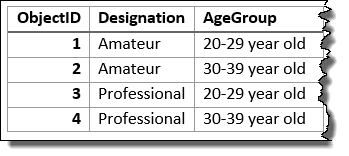The count statistic (for strings and numeric fields) counts the number of nonnull values. The count of the following values equals 5: [0, 1, 10, 5, null, 6] = 5. The count of this set of values equals 3: [Primary, Primary, Secondary, null] = 3.

## Parameters

ParameterExplanationData type

Input Layer

The point features for which density will be calculated.

Features

Fields (optional)

One or more fields used to summarize similar features. For example, if you choose a single field called PropertyType with the values of commercial and residential, all of the residential fields would be summarized together, with summary statistics calculated, and all of the commercial fields would be summarized together.

If more than one field is chosen, each unique combination of values would be summarized together with summary statistics calculated. For example, consider a first field PropertyType with the values of commercial and residential, and a second field Occupied with the values of Yes or No. There would be four possible combinations that could be summarized with summary statistics calculated.

String

Summary Fields (optional)

The statistics that will be calculated for specified fields. Different statistics are available depending if the specified field is a string, numeric, or date field.

The following are available statistics types:

• Any—This is a sample string from a field of type string.
• Count—Calculates the number of nonnull values. It can be used on numeric fields or strings. The count of [null, 0, 2] is 2.
• Count Distinct—Calculates the number of distinct, nonnull values. It can be used on numeric fields or strings. The count distinct result of [null, 4, 3, 4] is 2.
• Sum—The sum of numeric values in a field. The sum of [null, 1, 3] is 4.
• Sum Of Squares—The sum, over all observations, of the squared differences of each observation from the overall mean. The sum of squares of [null, 2.2, 3.1, 4.7] is 3.206.
• Min—The minimum value of a numeric field. The minimum of [0, 2, null] is 0.
• Max—The maximum value of a numeric field. The maximum value of [0, 2, null] is 2.
• Mean—The mean of numeric values. The mean of [0,2, null] is 1.
• Range—The range of a numeric field. This is calculated as the minimum value subtracted from the maximum value. The range of [0, null, 1] is 1. The range of [null, 4] is 0.
• Variance—The variance of a numeric field in a track. The variance of  is null. The variance of [null, 1,1,1] is 1.
• Standard Deviation—The standard deviation of a numeric field. The standard deviation of  is null. The standard deviation of [null, 1,1,1] is 1.

String

## Output layer

The output layer will be a table containing the fields provided in the Fields parameter, a count attribute of the number of features summarized by that record, and any summarized attributes as specified in the Summary Fields parameter.

## Considerations and limitations

• Inputs may be a tabular layer or a layer with geometry (points, lines, or areas).
• You can use this tool with spatial data, however, the result will be tabular. You can then join your results to spatial data using the Join Features tool.